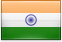#India publishes notification to amend target fuel consumption of N3 and M3 from January, 2021

On September 16, 2020, India’s Ministry of Power published a notification amending the equation for calculating fuel consumption targets for vehicles in the N3 category. As per the notification, from January 1, 2021 onwards, diesel vehicles of gross weight of 12 tonnes or more in M3 and N3 category that are in compliance with emission norms BS-IV shall be tested with respect to constant speed fuel consumption as prescribed by the Ministry of Roads and Transport until the Bureau of Indian Standards formulates the standards (see table below). The second phase standards, effective from April 1, 2021, as specified in the notification dated August 16, 2017, was repealed.

X：Gross vehicle weight in tonnes

Y：Normalized value (fuel consumption) in litres/100kms

Table 1(a) N3-Rigid vehicles at 40km/h

 Gross vehicle weight range Axle configuration Equation for deriving target fuel consumption (l/100km) 12.0 tonnes and above 4×2 Y=0.362X+10.327 16.2 tonnes and above 6×2 Y=0.603X+6.415 16.2 tonnes and above 6×4 Y=0.723X+4.482 25.0 tonnes and above 8×2 Y=0.527X+8.333 25.0 tonnes and above 8×4 Y=0.928X-0.658 31.0 tonnes and above 10×2 Y=0.960X-5.100

Table 1(b) N3-Rigid vehicles at 60km/h

 Gross vehicle weight range Axle configuration Equation for deriving target fuel consumption (l/100km) 12.0 tonnes and above 4×2 Y=0.788X+9.003 16.2 tonnes and above 6×2 Y=0.755X+9.546 16.2 tonnes and above 6×4 Y=1.151X+3.122 25.0 tonnes and above 8×2 Y=0.650X+12.160 25.0 tonnes and above 8×4 Y=0.968X+7.692 31.0 tonnes and above 10×2 Y=0.650X+12.160

Table 1(c) N3- Tractor Trailer vehicles at 40 km/h

 Gross vehicle weight range Axle configuration Equation for deriving target fuel consumption (l/100km) 35.2 tonnes and above 4×2 Y=0.986X-7.727 40.2 tonnes and above 6×2 Y=0.628X+6.648 40.2 tonnes and above 6×4 Y=1.255X-18.523

Table 1(d) N3- Tractor Trailer vehicles at 60 km/h

 Gross vehicle weight range Axle configuration Equation for deriving target fuel consumption (l/100km) 35.2 tonnes and above 4×2 Y=0.208X+32.198 40.2 tonnes and above 6×2 Y=0.628X+15.298 40.2 tonnes and above 6×4 Y=1.342X-13.390

Table 1(e) M3-Vehicles at 40 km/h

 Gross vehicle weight range Axle configuration Equation for deriving target fuel consumption (l/100km) 12.0 tonnes and above 4×2 and 6×2 Y=0.509X+11.062

Table 1(f) M3-Vehicles at 60 km/h

 Gross vehicle weight range Axle configuration Equation for deriving target fuel consumption (l/100km) 12.0 tonnes and above 4×2 and 6×2 Y=0.199X+19.342

The revised notification can be downloaded from the following URL.

http://egazette.nic.in/WriteReadData/2020/221869.pdf